Courses

# Quant Mock Test - 11

## 20 Questions MCQ Test Mock Test Series for CLAT 2020 | Quant Mock Test - 11

Description
This mock test of Quant Mock Test - 11 for CLAT helps you for every CLAT entrance exam. This contains 20 Multiple Choice Questions for CLAT Quant Mock Test - 11 (mcq) to study with solutions a complete question bank. The solved questions answers in this Quant Mock Test - 11 quiz give you a good mix of easy questions and tough questions. CLAT students definitely take this Quant Mock Test - 11 exercise for a better result in the exam. You can find other Quant Mock Test - 11 extra questions, long questions & short questions for CLAT on EduRev as well by searching above.
QUESTION: 1

### A train covers the distance from Delhi to Patna at the speed of 42 kmph and from Patna to Delhi at 48 kmph. What is the average speed of the train?

Solution: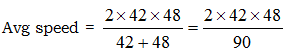= 44.8 km/h

QUESTION: 2

### The cost of 24 pens and 36 pencils is Rs 720. What is the cost of 6 pens and 9 pencils?

Solution:

24 Pen + 36 Pencil = 720
Divide by 4, we get 720/4 = 180

QUESTION: 3

### If the sum of five consecutive even numbers A, B, C, D and E is 110, what is the product of B and D?

Solution: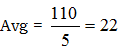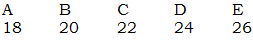Now, B x D = 480

QUESTION: 4

Two years ago the ratio of the ages of Manoj and Shyam was 15:11. Two years hence the ratio of their ages will be 17 : 13. What is the present age of Shyam?

Solution:

Ratio (2) = Actual (4 years)
1 = 2 yrs.
11 = 22 yrs
So present – age = 24 years.

QUESTION: 5

Pinky invested an amount of Rs 24500 at the rate of 9% per annum. After how many years will she get a simple interest of Rs 37485?

Solution: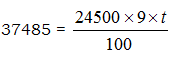So t = 17 yrs.

QUESTION: 6

50 persons can complete a piece of work in 50 days. How many persons with twice the efficiency will complete the same piece of work in 10 days?

Solution:

50 x 50 x 1 = 2x  x 10 x 1
So x = 125

QUESTION: 7

Two candidates fought an election. One of them got 60% of the total votes and won by 550 votes. If 5% of votes were declared invalid what was the total number of votes polled?

Solution:

Total = 100
Valid = 95
Winner = 60
Loser = 40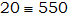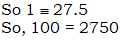QUESTION: 8

Two sums of money are proportional to 7:9. If the first one is Rs 140, what is the other?

Solution: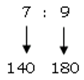QUESTION: 9

A man swims 21 km downstream and 14 km upstream, taking 7 hours each time. What is the speed of the stream?

Solution:

Speed downstream = 21/7 = 3km/h
Speed downstream = 14/7 = 2 km/h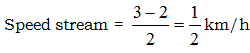QUESTION: 10

A 20m long and 16m wide rectangular hall is surrounded by a 2-metre wide verandah. Find the area of the verandah.

Solution:

Area = 24 x 18 = 20 x 16
= 432 – 320
= 112

QUESTION: 11

Two horses are sold for Rs 1955 each. The first one is sold at 15% profit and the other one at 15% loss. What is the total profit or loss?

Solution:

Loss% = 2.25%
S.P = 1955 x 2 = 3910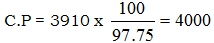Profit = 90

QUESTION: 12

A number when divided by 527 gives a remainder 31. What remainder will be obtained by dividing the same number by 17?

Solution: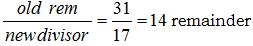QUESTION: 13

Directions: What should come in place of question mark (?) in the following equations?

Q.

14973 - ? – 1976 + 3688 = 15344

Solution:

14973 + 3688 – 15344 – 1976 = x
So x = 13431

QUESTION: 14

4379 x 777 = ?

Solution:
QUESTION: 15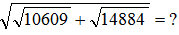Solution: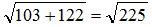QUESTION: 16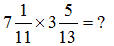Solution: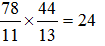QUESTION: 17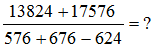Solution:

31400/628 = 50

QUESTION: 18

If 45% of a number is 1291.5, what is 67.5% of that number?

Solution: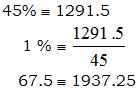QUESTION: 19

The cost of 19 kg Apples is Rs 1558, that of 17 kg Tomatoes is Rs 595, and that of 13 kg Oranges is Rs 949. What is the total cost of 11 kg Apples, 7 kg Tomatoes and 3 kg Oranges?

Solution:

11 ‘A’ + ‘T’ + 3 ‘OR’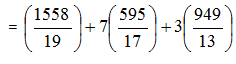= 902 + 245 + 219

QUESTION: 20

A number when multiplied by six times of itself gives value equal to 1176. What is the number?

Solution: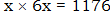x2 = 196
x = 14